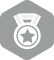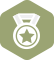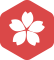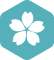### 发现古希腊和古罗马的神话很多神都一样，名字不一样，为什么？2007年7月 总版技术专家分月排行榜第二
2006年6月 总版技术专家分月排行榜第二2007年7月 MS-SQL Server大版内专家分月排行榜第一
2007年6月 MS-SQL Server大版内专家分月排行榜第一
2007年4月 MS-SQL Server大版内专家分月排行榜第一
2007年3月 MS-SQL Server大版内专家分月排行榜第一
2006年6月 MS-SQL Server大版内专家分月排行榜第一ustc_tweeg

vc++调用matlab函数

但是，由于Matlab开发平台的局限性，其上面开发的程序不能脱离Matlab运行环境，因而处理一些实际应用问题时显得灵活性不足，而VC++一定程度上能够弥补这一漏洞，因此，现将二者结合共用，各献其长，可以为...

C/C++ VS中调用matlab函数的方法

C/C++ VS中调用matlab函数的方法 Matlab数值等矩阵运算具有很大优势，并且Matlab提供了强大的工具箱可以使用，C/C++开发，我们可以VS下调用matlab函数进行混合编程。下面主要介绍VS中调用matlab函数的环境...

VC中调用matlab函数

python中调用matlab函数

matlab的很多函数有着强大的功能，python中调用matlab函数，无论是计算还是绘图都有巨大的前景，有时间会写写

VC++中调用MATLAB中的数据

Qt中调用MatlabEngine总结

Qt调用Matlab的方法： 1.调用matlab封装成的dll ...Qt调用MatlabEngine本质上是Qt打开Matlab平台。 首先，.pro文件配置好engine.h的头文件和 lib库文件。engine一般Matlab的extern文件夹

qt界面中调用matlab engine并嵌入Matlab cmd Window

VC++与Matlab混合编程之一：调用Matlab引擎

VC中调用MATLAB的，总结有以下几种方法：1、是调用Matlab引擎；2、是调用Matlab中M函数转化成的dll文件；3、调用Matlab中M函数转化成c语言的函数。  本节之讲解方法1，因为方法1最简单，只要配置好VC的相关...

VC中调用matlab函数 VC++与Matlab混合编程

Matlab是一款将数值分析、矩阵计算、信号处理和图形显示结合一起，包含大量高度集成的函数可供调用，适合科学研究、工程设计等众多学科领域使用的一种简洁、高效的编程工具。不过由于Matlab使用的是解释性语言，...

vs2015中调用matlab函数

D：Mat是matlab安装路径包含目录：D:\Mat\extern\include;D:\Mat\extern\include\win32;库目录：D:\Mat\extern\lib\win32\microsoftD:\Mat\extern\lib\win32链接器：常规 附加库目录D:\Mat\bin\win32链接器：输入...

c++调用matlab engine方法

@author:Donald-Hu @theme:c++调用matlab engine方法 @time:2016/8/10 #include #include #include "engine.h" using namespace std; void main() { const int N = 50; double x[N], y[N]; int j = 1; for ...

vc++调用matlab

Matlab是一款将数值分析、矩阵计算、信号处理和图形显示结合一起，包含大量高度集成的函数可供调用，适合科学研究、工程设计等众多学科领域使用的一种简洁、高效的编程工具。不过由于Matlab使用的是解释性语言，...

python 调用matlab中函数/方法

https://ww2.mathworks.cn/help/matlab/matlab_external/install-the-matlab-engine-for-python.html 下面演示具体步骤： 1、安装matlab和python 如果已经安装了matlab和python，这一步就可以跳过了。 2、给...

C++ MATLAB 混合编程——VS项目调用MATLAB函数

[Matlab]VS和Matlab混合编程(调用Matlab Engine)

matlab engine: Codeblocks使用C++调用matlab

VS通过调用matlab引擎方式VS里调用matlab函数和自己写的.m文件

MATLAB调用Python Python调用MATLAB 将MATLAB代码打包成组件然后Python集成

Python中调用MATLAB函数的实现与问题

c 调用 matlab engine 自定义函数

Tools/Projects and Solutions/VC ++ Directories Inlcude files: C:/Program Files/MATLAB/R2009a/extern/include Library files: C:/Program Files/MATLAB/R2009a/extern/lib2. Property

Python 调用Matlab函数或者调用M文件方法

Method for calling the MATLAB function from Python import numpy as np import scipy.io as sio import toollib import matlab.engine eng = matlab.engine.start_matlab() data_m = sio.loadmat('matlab1.ma...

Linux下c++调用自己编写的matlab函数：通过matlab引擎实现

Android开发精典案例60个【源码】

60个Android开发精典案例，好东西 - 给大家分享60个Android开发的精典案例，包含任务监听、设备适配，游戏框架搭建，特效实现，多点触控，网络协议，游戏关卡设置等内容。特别是做游戏开发的朋友值得研究。喜欢就拿走吧！